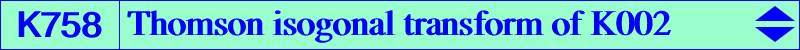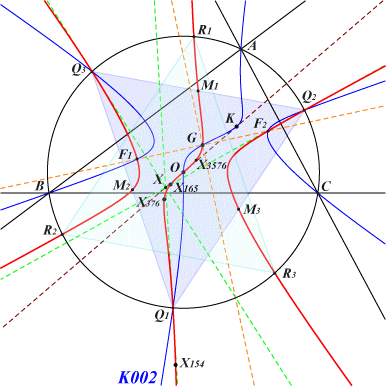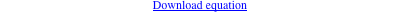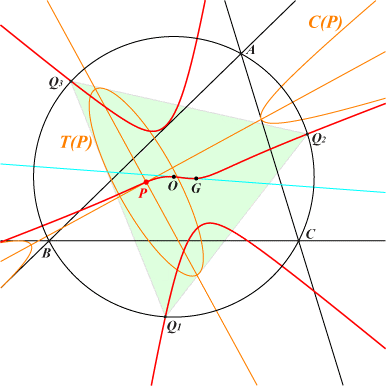too complicated to be written here. Click on the link to download a text file.X(2), X(3), X(154), X(165), X(376), X(3576) four foci of the Steiner inellipse Q1, Q2, Q3 : vertices of the Thomson triangle M1, M2, M3 : midpoints of the Thomson triangle reflections about X(3) of these ten latter points more generally, images under h(X3, ±1/3) of the points on K004The Thomson cubic K002 is inscribed in the Thomson triangle (T) hence its isogonal transform with respect to (T) is also a circum-cubic of (T) namely K758. K758 is a central cubic with center X(3) and the inflexional tangent at this point is the Brocard axis. It has three real asymptotes which are the perpendicular bisectors of ABC. These properties also hold for the Darboux cubic K004 since K758 is the homothetic of K004 under h(X3, ±1/3). It follows that K004 contains the images of the four foci of the Steiner inellipse under h(X3, 3) and their reflections about (X3) which are also the images under h(X4, 3). See other similar cubics in CL065. Hence K758 and K004 define a pencil of cubics which must contain the two degenerate cubics : • union of the perpendicular bisectors of ABC, • union of the Brocard axis and the line at infinity counted twice. Since the centroid X(3524) of (T) doesn't lie on the cubic, K758 cannot be a pK in (T) but only a psK with pseudo-pivot X(3524). The tangents at the vertices of (T) concur at the pseudo-isopivot X, obviously not on the cubic. X is the homothetic of X(6) under h(X3, -1/3), X(31884) in ETC, with SEARCH number 8.71218102723990.Consider two conics C(P) and T(P) with same center P inscribed in the reference triangle ABC and in the Thomson triangle (T) respectively. These conics have the same axes if and only if P lies on K758. When P = X2, C(P) and T(P) are both the Steiner inellipse since it is inscribed in both triangles. The figure is obtained when P = X(6194).K758 and K002 have nine known common points namely X(2), X(3), the four foci of the Steiner inellipse, Q1, Q2, Q3. They generate a pencil of cubics passing through these same points which contains the focal cubic K463, the central stelloid K833 and its Thomson isogonal transform K727. See Table 81. *** Another remarkable pencil is generated by K758 and the decomposed cubic which is the union of the Euler line and the circumcircle. Every cubic is a central cubic with center O, passing through G, X(376), Q1, Q2, Q3 and their reflections R1, R2, R3 in O. K764, K1260, K1261 are other members of this pencil. *** A locus property (Angel Montesdeoca, 2018-07-09) Let P be a point with cevian triangle PaPbPc. Ac, Ab are the midpoints of BPc, CPb respectively and A' is the circum-center of triangle AAbAc. B' and C' are defined likewise. ABC and A'B'C' are perspective if and only if P lies on the line at infinity or on the Steiner circum-ellipse or on the Lucas cubic K007. In this latter case, the triangles are homothetic and the center of homothety lies on K758. See further details and a generalization here. *** See the analogous cubic K1327, homothetic of K758 under h(G, 2).